MORE IN Circuits and Transmission Lines
MU Electronics and Telecom Engineering (Semester 3)
Circuits and Transmission Lines
May 2012
Total marks: --
Total time: --
INSTRUCTIONS
(1) Assume appropriate data and state your reasons
(2) Marks are given to the right of every question
(3) Draw neat diagrams wherever necessary

Attempt any FOUR from the following
1 (a) How many trees are possible for the given graph :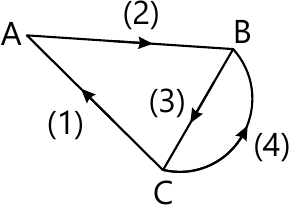5 M
1 (b) By mesh analysis determine the current through 2Ω resistor :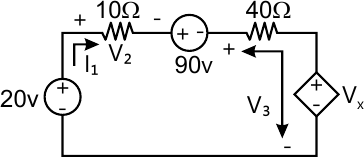5 M
1 (c) Find the condition of reciprocity for Z parameters
5 M
1 (d) Find I, in the circuit if dependent voltage source is
(i) 2V2
(ii) 1.5V35 M

2 (a) Find the current through 5Ω resistor. :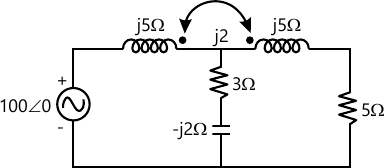10 M
2 (b) Find the Network function $\frac{V_1}{I_1}, \frac{V_2}{V_1} \ and \ \frac{V_2}{I_1}$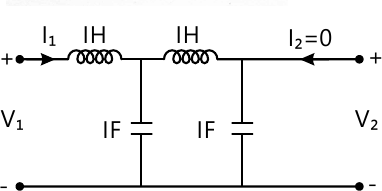10 M

3 (a) The switch is changed from position 1 to position 2 at t=0, Steady state having reached before switching. Find values of
$i, \frac{di}{dt} \ and \ \frac{d^2i}{dt^2} \ and \ t=0^+$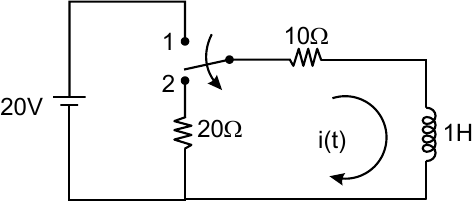10 M
3 (b) In the network, the switch is opened at t=0. Find i(t).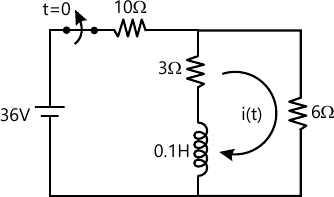10 M

4 (a) Write down the tieset matrix & obtain the network equilibrium equation in matrix form using KVL. Calculate loop currents: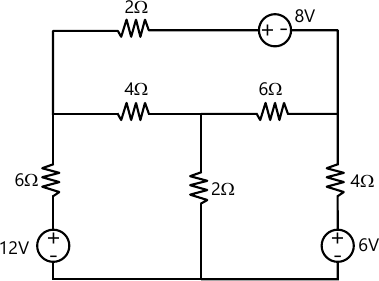10 M
4 (b) Determine Y & Z parameters for the network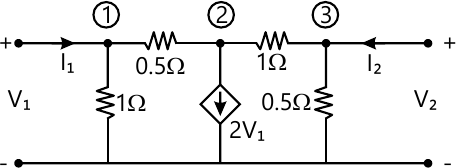10 M

5 (a) Synthesize the following function $Z\left(s \right)= \frac{6\left(s+2 \right)\left(s+4 \right)}{s\left(s+3 \right)}$
Use Foster -II Method.
8 M
5 (b) A driving point R-L admittance function is given by-
$y_{RL}\left(s \right)= \frac{s^2+6s+8}{s^2+4s+3}$
Use cauer - I Method
6 M
5 (c) Synthesize the following YRL(s) using cauer II from
$Y_{RL}\left(s \right)= \frac{\left(s+1 \right)\left(s+4 \right)}{s\left(3s+4 \right)}$
6 M

6 (a) Find the current I in the network, using superposition theorem.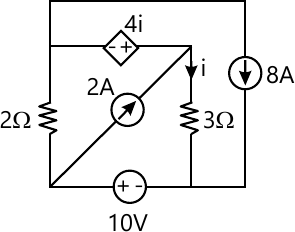10 M
6 (b) (i) Check the given polynomial for Hurwitz
$P\left(s \right)=s^5+8s^4+24s^3+28s^2+23s+6$
5 M
6 (b) (ii) Test whether $F\left(s \right)= \frac{{5(s+1)}^2}{s^3+2s^2+2s+40}$ is positive real function.
5 M

7 (a) Find the current through 10Ω resistor (Thevenin's theorem).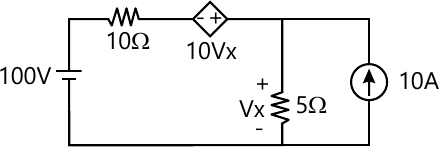(b) Determine the hybrid parameter of the network.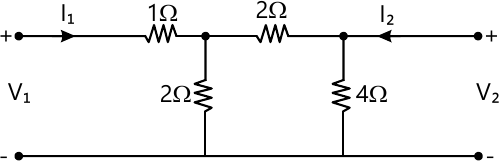20 M

More question papers from Circuits and Transmission Lines